# University of Washington, CSE 142

## Lab 5: Strings, cumulative algorithms, while loops, fencepost loops

Except where otherwise noted, the contents of this document are Copyright 2013 Stuart Reges and Marty Stepp.

lab document created by Marty Stepp, Stuart Reges and Whitaker Brand

# Basic lab instructions

• Mouse over if you're not sure what they mean!
• Talk to your classmates for help.
• You may want to bring your textbook to future labs to look up syntax and examples.
• Stuck? Confused? Have a question? Ask a TA for help, or look at the book or past lecture slides.
• Complete as much of the lab as you can within the allotted time. You don't need to keep working on these exercises after you leave.
• Feel free to complete problems in any order.
• Make sure you've signed in on the sign-in sheet before you leave!

# Today's lab

Goals for today:

• practice using `String` methods
• practice cumulative algorithms
• exposure to fencepost and sentinel loop patterns
• use `while` loops for indefinite repetition

# `String` methods

Method name Type Returns....
`charAt(index)` `char` the character at the given index
`indexOf(str)` `int` the index where the start of the given `String` appears in this string (or -1 if not found)
`length()` `int` the number of characters in this `String`
`replace(str1, str2)` `String` a new string with all occurrences of str1 changed to str2
`substring(index1, index2)`
or `substring(index1)`
`String` the characters in this string from index1 (inclusive) to index2 (exclusive); if index2 is omitted, grabs till end of string
`toLowerCase()` `String` a new string with all lowercase letters
`toUpperCase()` `String` a new string with all uppercase letters

# Exercise : String expressions

Write the results of each expression with `String`s in "quotes" and characters in 'single quotes'.
Hint: `String`s index starting at 0. A `String` with 10 characters has the indices 0-9!

```//       index 0123456789012345
String str1 = "Frodo Baggins";
String str2 = "Gandalf the GRAY";
```
 `str1.length()` `13` `str2.charAt(0)` `'G'` `str1.indexOf("o")` `2` `str2.toUpperCase()` `"GANDALF THE GRAY"` `str1.substring(4)` `"o Baggins"` `str2.substring(3, 14)` `"dalf the GR"` `str2.replace("a", "oo")` `"Goondoolf the GRAY"`

# Exercise : Catching `String` method returns

Write the value of the variable as prompted. Put `String`s in "quotes" and characters in 'single quotes'.

```//       index 0123456789012345
String str1 = "Frodo Baggins";
String str2 = "Gandalf the GRAY";
```
 `String str3 = str2.replace("gray", "white")` // str3    = ? `"Gandalf the GRAY"` `str2.toLowerCase()` // str2    = ? `"Gandalf the GRAY"` `str2 = str2.toLowerCase()` // str2    = ? `"gandalf the gray"` `int index = str1.toLowerCase().indexOf("B")` // index = ? `-1`

# Exercise : String Equality

Recall that although we use `==` to compare the values of primitive types, such as `ints, doubles,` and `chars`. However, `==` has a different meaning for `Strings`: it evaluates to `true` when one String is set to equal another (ex.` String a = b`). To see whether two Strings have the same value, we instead use `.equals()`.

Enter `true` or `false` for the following:

 ```String a = "hello"; String b = "hel" + "lo";``` // a == b?          `false` ```String a = "hello"; String b = "hel" + "lo";``` // a.equals(b)? `true` ```String a = "world"; String b = a;``` // a == b?          `true` ```String a = "world"; String b = a;``` // a.equals(b)? `true`

# Exercise : equalsIgnoreCase()

`equalsIgnoreCase()` is identical to `.equals()`, except it ignores the case of the `String`s being compared.
Enter `true` or `false` for the following:

 `"abc".equalsIgnoreCase("abc");` `true` `"abc".equalsIgnoreCase("ABC");` `true` `"abc".equals("ABC");` `false` `"iLoveHalloween!".equalsIgnoreCase("iLoVeHaLlOwEeN!");` `true`

# Exercise : ProcessName

Copy/paste the following code into jGRASP, or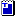download it. Then go to the next slide.

```import java.util.*;  // for Scanner

public class ProcessName {
public static void main(String[] args) {
Scanner console = new Scanner(System.in);

// read the entire input as a single line
String input = console.nextLine();

System.out.println("Your name is: " + name);
}
}
```

continued on the next slide ...

# Exercise - code to add

Add code to the program so that it reads the user's first and last name (reading the entire line of input as a single string), then prints the last name followed by a comma and the first initial. Assume that the user types a single first name, a space, and then a single last name.

Example:
```Type your name: Jessica Miller
```
If you get stuck, ask a TA for help!

# Exercise : ProcessName Solution

```import java.util.*;  // for Scanner

public class ProcessName {
public static void main(String[] args) {
Scanner console = new Scanner(System.in);

// read the entire input as a single line
String input = console.nextLine();

int spaceIndex = input.indexOf(" ");
String lastName = input.substring(spaceIndex + 1);
char firstInitial = input.charAt(0);

String name = lastName + ", " + firstInitial + ".";

System.out.println("Your name is: " + name);
}
}```

# Cumulative algorithms

A cumulative algorithm involves incrementally accumulating a value by repeatedly adding, multiplying, dividing, etc., while storing the result in a variable.

Key aspects of a cumulative algorithm: A loop, and a variable declared outside the loop whose value is modified inside the loop.

Example: Cumulative algorithm to sum the numbers 1-100:
```int sum = 0;                // safe default value, 0 doesn't affect a sum
for (int i = 1; i <= 100; i++) {
sum = sum + i;
}
System.out.println(sum);    // 5050
```

# Exercise : repl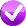Write a method named `repl` that accepts a `String` and a number of repetitions as parameters and returns the `String` concatenated that many times. For example, the call

``repl("hello", 3)``
returns
``"hellohellohello"``
If the number of repetitions is 0 or fewer, an empty string is returned.

(Hint: This is best solved with a cumulative algorithm. Start with an empty string and build it up piece by piece.)

# Exercise : Getting textbook code files

In this exercise we will download a program from the textbook for a debugging exercise.

1. Go to the course web page and click the Textbook sidebar link.
2. Find the section labeled Code Files and click the "code files" link. It's kind of hard to see, but in the sentence "You can access a full set of..." you'll see the text "code files" and you'll want to click on that.
3. Click on the 4ed link. This will bring you to a listing of all chapters. Click the link for ch04.
4. Download and save `Hailstone.java`. Right-click the file name and choose the option to Save the Link in the folder you have been using for lab work.
5. Compile and run `Hailstone.java` in jGRASP.

# Exercise : jGRASP Debugger

We are going to practice using the jGRASP debugger with `Hailstone.java`. This program computes a sequence of integers called a hailstone sequence. (This is related to an unsolved problem in mathematics known as the Collatz Conjecture.)

• Recall: To Set a breakpoint, move your cursor to the beginning of the desired line until you see a stop-sign icon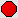and click.
• Recall: To run a program in debug mode, click the debug icon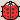(looks like a ladybug).
• Recall: To resume a stopped program, press the top-left play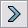or step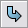buttons.

continued on the next slide...

# Exercise - Values of `value`

• For this exercise you will trace the first call: `printHailstoneMaxMin(7, 10);`
• Set a breakpointthat will allow you to determine the first several distinct values of the variable `value` as the loop executes.
# `value`
first value `7`
second value `22`
third value
`11`
fourth value
`34`
fifth value
`17`
sixth value
`52`

continued on the next slide...

# Exercise - Values of `min`

• Now you will trace the second call: `printHailstoneMaxMin(7, 20);`
• Clear your previous breakpoint by clicking on it a second time, then set a new breakpoint for the second method call, and resume execution.
• Use your new breakpoint to determine the first several distinct values of `min` during the second call. You may have to step through several iterations until you get to a distinct value of `min`.
# `min`
first value `7`
second value
`5`
third value
`4`
fourth value
`2`
fifth value
`1`

# Fencepost Loops

A fencepost loop is a common algorithmic pattern where you want to perform N tasks with N-1 things between them. It's like a fence with N posts with N-1 wires between the posts.

To achieve this, place one "post" outside your loop, then alternate between "wires" and "posts" inside the loop.

Example:

```System.out.print(1);                 // |==|==|==|==| fence
for (int i = 2; i <= 5; i++) {
System.out.print(".." + i);      // 1..2..3..4..5
}
```

# Exercise : printLettersWrite a method named `printLetters` that takes a `String` as its parameter and that prints the letters of the String, separated by dashes. For example, the call of `printLetters("Rabbit")` should print:
```R-a-b-b-i-t
```
(Hint: This is a fencepost problem. Remember that each `String` object has a `length` method that tells you how many characters are in the `String` and a `charAt` method that gets you individual characters of the `String`.)

# `while` Loops

`for` loops are fantastic for when we know how many times we want to repeat something. But sometimes we won't know how many times we'll want to repeat something in advance! `while` loops repeat indefinitely while a given condition is true.

```while (test) {
statement(s);
}
```

Example:

```int num = 1;
while (num < 5) {
System.out.print(num + " ");     // output: 1 2 3 4
num++;
}
```

How many times does "Wrong! Try again:" print for the following user inputs?
 ```import java.util.*; // lets us use the Scanner public class Password { public static void main(String[] args) { Scanner console = new Scanner(System.in); String password = "password"; // secure System.out.print("Enter your password: "); String input = console.next(); while (!input.equals(password)) { System.out.print("Wrong! Try again: "); input = console.next(); } System.out.println("Welcome to DefCon!"); } }``` input: `password` 0 input: `hello?   abc123   password` 2 input: `pswd   PASS   word?   sm39!#2   password` 4

# Exercise : `while` loop basics

Consider the following loop.

```int x = 1;
System.out.print(x);
while (x < 100) {
x = x + x;
System.out.print(", " + x);
}
```
 How many times does the code in the `while` loop execute? 7 What output is produced by the overall code? 1, 2, 4, 8, 16, 32, 64, 128

# Exercise : `while` loop mystery

Fill in the boxes at right with the output produced by each method call.

```public static void mystery(int x) {
int y = 4;
while (y <= x) {
y = y + 4;
}
System.out.println(y);
}
```
 `mystery(1);` `4` `mystery(6);` `8` `mystery(19);` `20` `mystery(40);` `44`

# Exercise : `while` loop mysteryFill in the boxes at right with the output produced by each method call.

```public static void mystery(int x) {
int y = 1;
int z = 0;
while (2 * y <= x) {
y = y * 2;
z++;
}
System.out.println(y + " " + z);
}
```
 `mystery(1);` `1 0` `mystery(6);` `4 2` `mystery(19);` `16 4` `mystery(39);` `32 5` `mystery(74);` `64 6`

# Exercise : ProcessName2Modify your previous `ProcessName` program so that it re-prompts until the user types a name that is at least 5 letters total in length and has at least one space in it. Example:

```Type your name: Joe
Error, must be at least 5 chars with a space.
Error, must be at least 5 chars with a space.
Error, must be at least 5 chars with a space.
```

# Checkpoint: Congratulations!

Nice job making it this far--labs are tough!

These next problems get a little more challenging as we explore earlier concepts further.

We put a lot of problems in here so that you have plenty to refer back to later when working on homework. Don't feel bad if you don't finish all of them--Brett can't finish them all in a 50 minute lab, either! :)# Exercise : Syntax errors

The following Java program has several errors. Can you find them all?
 ``` 1 2 3 4 5 6 7 8 9 10 11 12 13 14 15 16 17 18 ``` ```public class StringOops { public static void main(String[] args) { Scanner console = new Scanner(System.in); System.out.print("Type your name: "); String name = console.nextString(); process(name); } public static void process(string "name") { if (name == Brett) { System.out.println("You must be really awesome."); } replace("a", "e"); toUppercase(name); name.substring(0, 3); System.out.println(name + " has " + name.length + " letters"); } } ```
Copy and paste the code into jGrasp and see if you can fix the errors.

# Exercise - Corrected version

```public class StringOops {
public static void main(String[] args) {
Scanner console = new Scanner(System.in);
String name = console.nextString();
process(name);
}

public static void process(String "name") {
if (name.equals("Brett")) {
System.out.println("You must be really awesome.");
}
name = name.replace("a", "e");
name = name.toUpperCase();
name = name.substring(0, 3);
System.out.println(name + " has " + name.length() + " letters");
}
}
```

# Exercise : `while` loop mysteryFill in the boxes at right with the output produced by each method call.

```public static void mystery2(int x, int y) {
int z = 0;
while (x % y != 0) {
x = x / y;
z++;
System.out.print(x + ", ");
}

System.out.println(z);
}
```
 `mystery2(25, 2);` `12, 1` `mystery2(32, 4);` `0` `mystery2(10345, 10);` `1034, 103, 10, 3` `mystery2(63, 2);` `31, 15, 7, 3, 1, 0, 6`

# Exercise : digitSumWrite a method named `digitSum` that accepts an integer as a parameter and returns the sum of the digits of that number. For example, the call `digitSum(29107)` returns 2+9+1+0+7 or 19. For negative numbers, return the same value that would result if the number were positive. For example, `digitSum(-456)` returns 4+5+6 or 15. The call `digitSum(0)` returns 0.

(Hint: This is a cumulative algorithm. To extract a digit from a number, use `/ 10` and `% 10` operations.)

# Exercise : longestNameWrite a method named `longestName` that reads names typed by the user and prints the longest name (the name that contains the most characters) in the format shown below. Your method should accept a console `Scanner` and an integer n as parameters and should then prompt for n names.

A sample execution of the call `longestName(console, 4)` might look like the following:

```name #1? roy
name #2? DANE
name #3? sTeFaNiE
name #4? Erik
Stefanie's name is longest
```

Try to solve this problem in Practice-It: click on the check-mark above!

# Exercise : printFactorsWrite a method called `printFactors` that accepts an integer as its parameter and that prints the factors of that number, separated by the word `"and"`. Recall that a factor is a number that goes evenly into another number. For example, the call `printFactors(24)` should print the following output:

```1 and 2 and 3 and 4 and 6 and 8 and 12 and 24
```

You may assume that the parameter value passed is greater than 0.

# If you finish them all...

If you finish all the exercises, try out our Practice-It web tool. It lets you solve Java problems from our Building Java Programs textbook.

You can view an exercise, type a solution, and submit it to see if you have solved it correctly.

Choose some problems from the book and try to solve them!